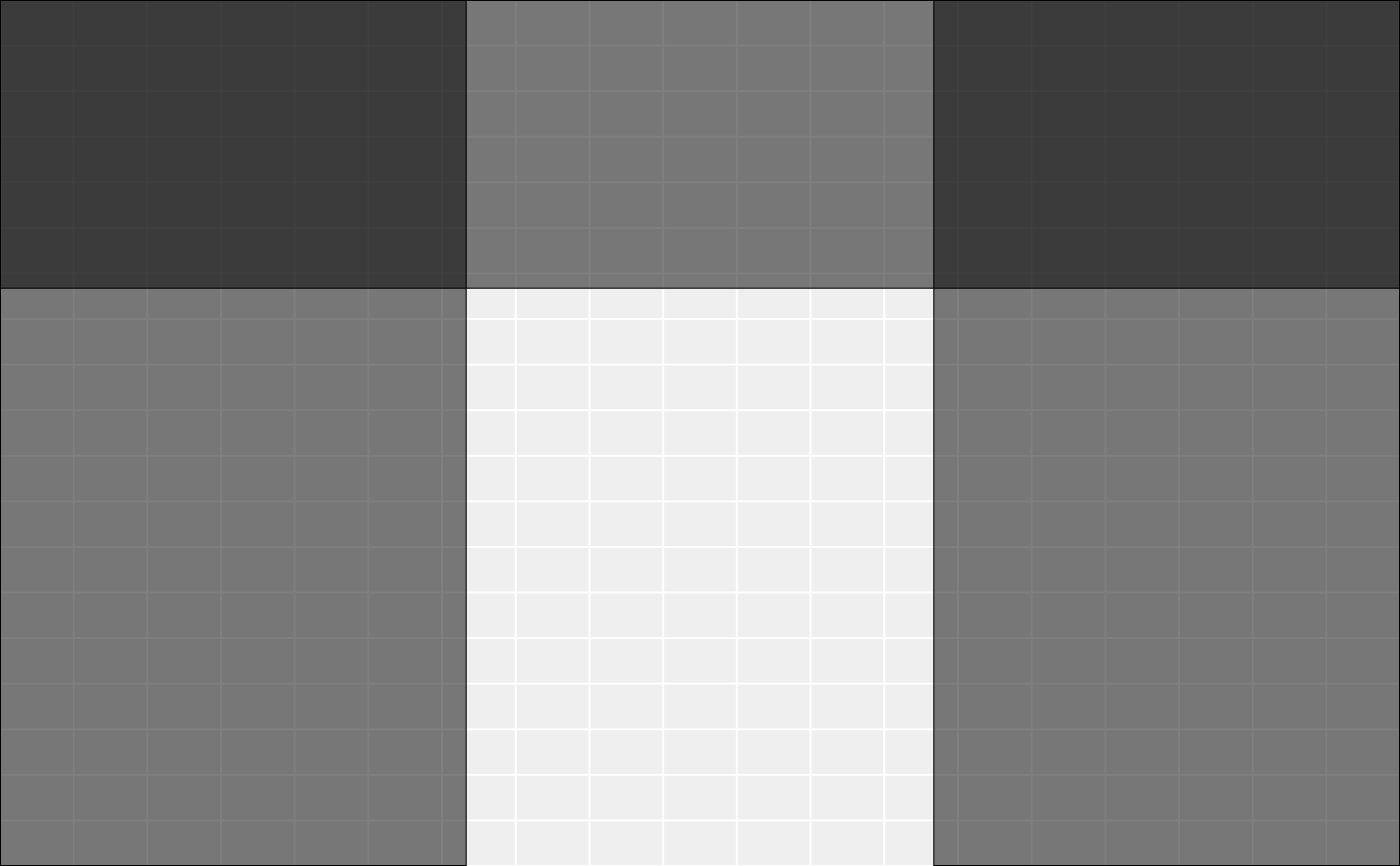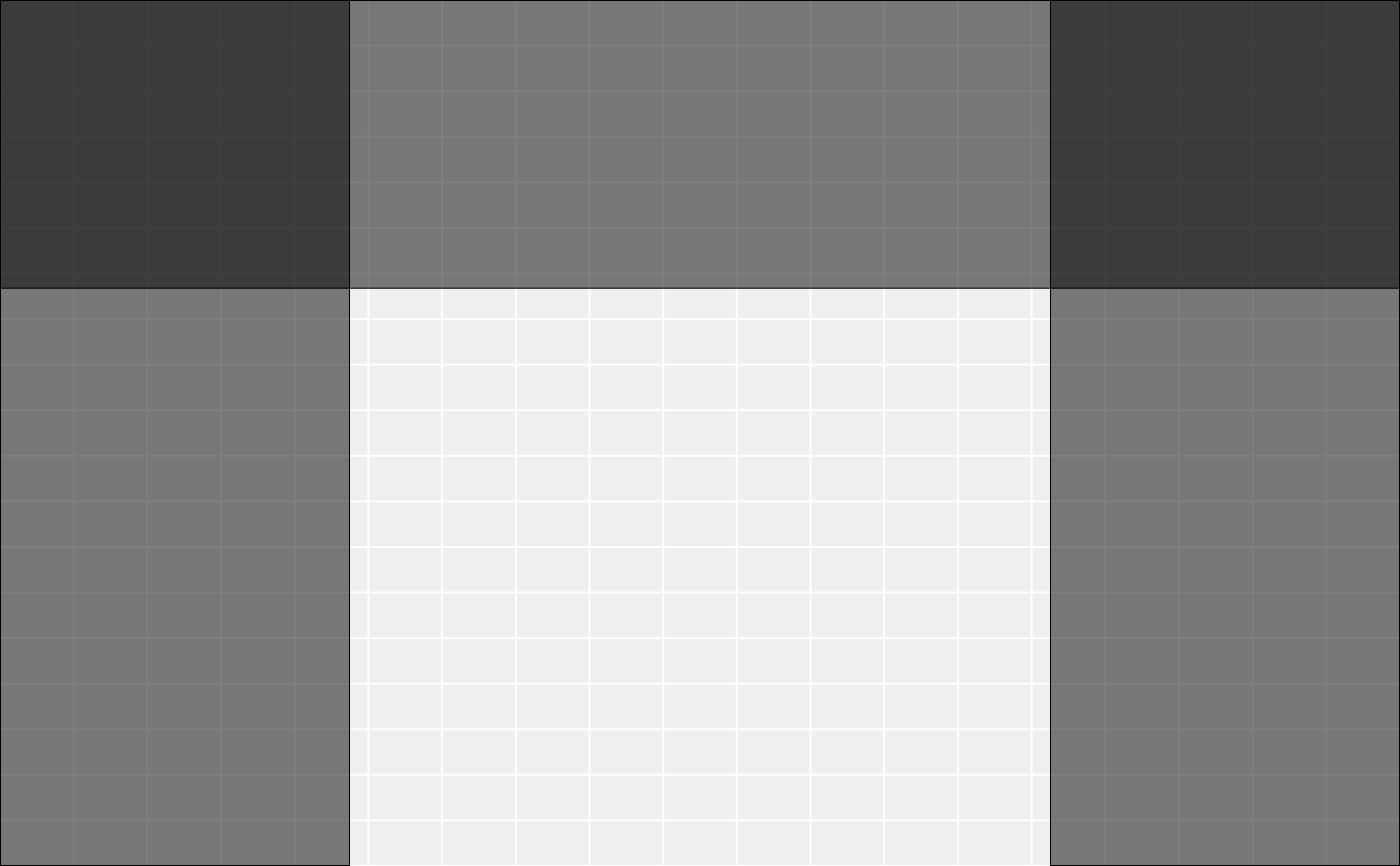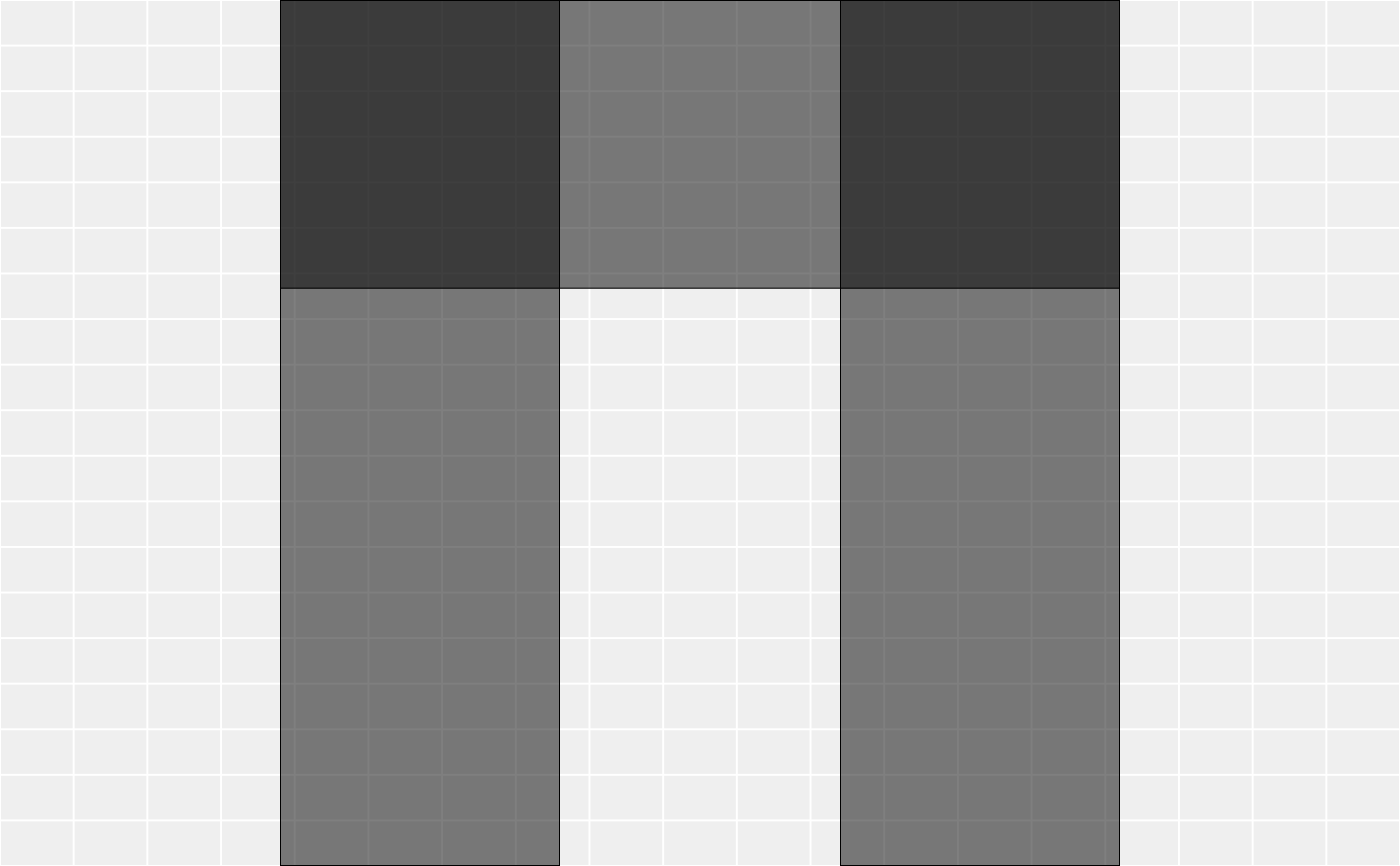Insert new columns in a gtable and adjust the grob placement accordingly. If columns are added in the middle of a grob spanning multiple columns, the grob will continue to span them all. If a column is added to the left or right of a grob, the grob will not span the new column(s).

gtable_add_cols(x, widths, pos = -1)

## Arguments

x

a gtable() object

widths

a unit vector giving the widths of the new columns

pos

new columns will be added to the right of this position. Defaults to adding col on right. 0 adds on the left.

## Value

A gtable with the new columns added.

Other gtable manipulation: gtable_add_grob(), gtable_add_padding(), gtable_add_rows(), gtable_add_space, gtable_filter()

## Examples

library(grid)
rect <- rectGrob(gp = gpar(fill = "#00000080"))
tab <- gtable(unit(rep(1, 3), "null"), unit(rep(1, 3), "null"))
tab <- gtable_add_grob(tab, rect, t = 1, l = 1, r = 3)
tab <- gtable_add_grob(tab, rect, t = 1, b = 3, l = 1)
tab <- gtable_add_grob(tab, rect, t = 1, b = 3, l = 3)
dim(tab)
#>  3 3
plot(tab)# Grobs will continue to span over new rows if added in the middle
tab2 <- gtable_add_cols(tab, unit(1, "null"), 1)
dim(tab2)
#>  3 4
plot(tab2)# But not when added to left (0) or right (-1, the default)